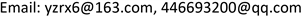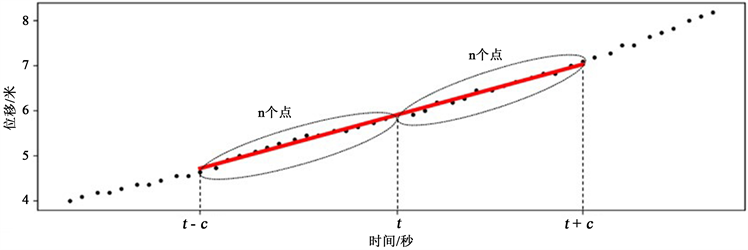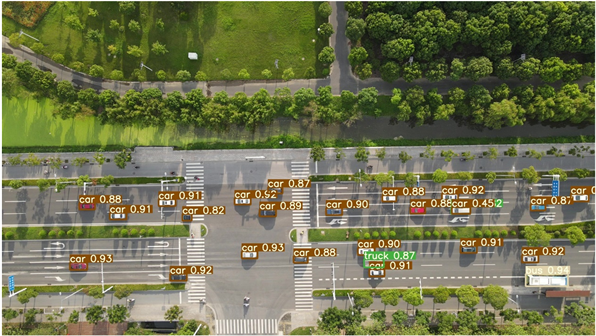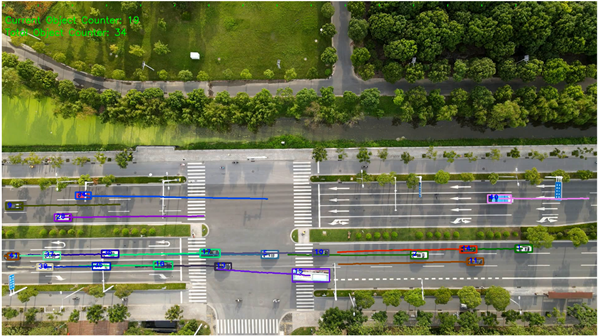﻿ 基于航拍视频的车辆速度估算研究 Research of Vehicle Speed Estimation Based on UAV Videos

Open Journal of Transportation Technologies
Vol. 10  No. 02 ( 2021 ), Article ID: 40920 , 7 pages
10.12677/OJTT.2021.102009Research of Vehicle Speed Estimation Based on UAV Videos

Shejun Deng, Yucheng Tang

Yangzhou University, Yangzhou JiangsuReceived: Jan. 11th, 2021; accepted: Mar. 5th, 2021; published: Mar. 12th, 2021ABSTRACT

With the rapid development of new technology, vehicle speed estimation based on video has attracted wide attention from researchers. However, there are still many challenges in extracting speed information from UAV videos, including the low accuracy of vehicle identification, the poor tracking effect, the lens jitter. In this paper, we propose a method for vehicle speed estimation. Firstly, we build a traffic dataset based on UAV for specific height, and obtain weight file through the deep-learning algorithm, and then obtain the trajectory through YOLO algorithm and Deep-sort algorithm. Thereafter, we propose a method to estimation vehicle speed based on linear regression algorithm. The result of experiments shows that the strategy has stronger anti-jamming ability and higher precision.

Keywords:Speed Estimation, Traffic Information Collection, Intelligent Transportation, UAV1. 引言

1) 城市道路中车辆数目较大，需避免车辆之间的相互影响提高车辆的检测与追踪的精度；

2) 镜头抖动等问题对车速的检测带来了一定的影响。

2. 基于航拍视频的车速检测Figure 1. Vehicle speed detection

2.1. 目标车辆检测

YOLO目标检测算法是端到端的算法，基本实现思路如下：

1) 将图像分为S S个网格，当某个检测目标中心落在所述网格中，则该网格负责预测该目标。

2) 在每个网格中预测B个边界框及对应的置信度。边界框包含5个参数中心点坐标，宽度，高度及置信度。

3) 在每个网格中预测C个目标类别的概率。

4) 最后在测试阶段将目标类别概率与置信度相乘，计算出每个目标框的特定类别的可能性，并通过阈值筛选去除多余检测框。

2.2. 目标车辆追踪

2016年Bewley A等提出了Deep-sort  多目标跟踪算法，该算法利用深度学习的思想，主要通过卷积神经网络提取特征，在跟踪过程中使用递归卡尔曼滤波预测的状态与新到达的状态之间的马氏距离和余弦距离这两个组合指标与阈值之间的关系进行关联度量，确保目标被遮挡后再次出现时仍然能匹配正确的ID，减少了ID的切换，达到了持续跟踪的目的。本文将YOLO的检测结果作为输入传给Deep-sort算法，实现目标车辆的追踪并输出车辆轨迹数据。

2.3. 车速计算

2.3.1. 坐标转换

$\left\{\begin{array}{l}x={N}_{x}{x}^{\prime }\\ y={N}_{y}{y}^{\prime }\end{array}$ (1)

2.3.2. 速度的计算Figure 2. Interval of value

t时刻车辆的瞬时速度求解公式见式2~4。

${v}_{x}=\frac{{\sum }_{i=1}^{m}\left({x}_{i}-\stackrel{¯}{x}\right)\left({t}_{i}-\stackrel{¯}{t}\right)}{{\sum }_{i=1}^{m}{\left({t}_{i}-\stackrel{¯}{t}\right)}^{2}}$ (2)

${v}_{y}=\frac{{\sum }_{i=1}^{m}\left({y}_{i}-\stackrel{¯}{y}\right)\left({t}_{i}-\stackrel{¯}{t}\right)}{{\sum }_{i=1}^{m}{\left({t}_{i}-\stackrel{¯}{t}\right)}^{2}}$ (3)

${v}_{t}=\sqrt{{v}_{x}^{2}+{v}_{y}^{2}}$ (4)

3. 实验结果

3.1. 数据采集

3.2. 数据集制作

3.3. 目标检测Figure 3. The result of car detection

3.4. 车辆跟踪

3.5. 车辆速度计算Figure 4. The result of trackingFigure 5. Vehicle speed estimation

$\epsilon =|\frac{{v}_{估}-{v}_{真}}{{v}_{真}}|×100%$ (5)

$\Phi =\frac{{\sum }_{i=1}^{n}{\epsilon }_{i}}{n}×100%$ (6)

4. 结论

Research of Vehicle Speed Estimation Based on UAV Videos[J]. 交通技术, 2021, 10(02): 71-77. https://doi.org/10.12677/OJTT.2021.102009

1. 1. 梁琳. 浅谈我国智能交通系统的发展[J]. 计算机与信息技术, 2008(10): 58-59.

2. 2. Lai, A.H.S. and Yung, N.H.C. (2000) Vehicle-Type Identification through Automated Virtual Loop Assignment and Block-Based Direction-Biased Motion Estimation. Intelligent Transportation Systems, 1, 86-97. https://doi.org/10.1109/6979.880965

3. 3. Pornpanomchai, C. (2009) Vehicle Speed Detection System. Signal and Image Processing Applications, Kuala Lumpur, 18-19 November 2009, 135-139. https://doi.org/10.1109/ICSIPA.2009.5478629

4. 4. 谭中慧. 基于视频的交通检测系统研究[D]: [硕士学位论文]. 西安: 长安大学, 2010.

5. 5. 赵晋, 龙浩, 张雅婷. 基于无人机航拍影像处理的车速检测研究[J]. 物联网技术, 2020(1): 16-23.

6. 6. 冼允廷. 基于视频图像分析的交通事件检测算法研究[D]: [硕士学位论文]. 南京: 东南大学, 2009.

7. 7. Bewley, A., Ge, Z., Ott, L., et al. (2016) Simple Online and Realtime Tracking. IEEE International Conference on Image Processing, Phoenix, 25-28 September 2016, 3464-3468. https://doi.org/10.1109/ICIP.2016.7533003